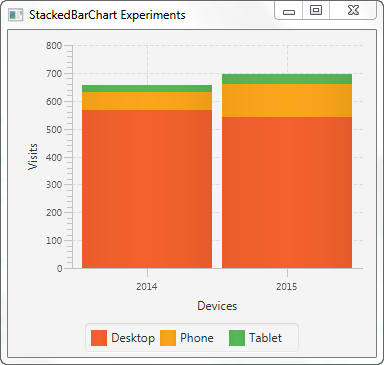# JavaFX StackedBarChart

 Jakob Jenkov Last update: 2016-05-27

The JavaFX StackedBarChart component is capable of drawing stacked bar charts inside your JavaFX applications. This can be useful in dashboard-like applications. The JavaFX StackedBarChart component is represented by the `javafx.scene.chart.StackedBarChart` class.

The `StackedBarChart` component is similar in function to the JavaFX BarChart component, but there are a few important differences. I will emphasize these differences when I get to them.

## StackedBarChart X Axis and Y Axis

A JavaFX StackedBarChart draws a stacked bar chart. A stacked bar chart is a two-dimensional graph, meaning the graph has an X axis and a Y axis. For stacked bar charts the X axis is typically a category of some kind, and the Y axis is numerical.

For instance, imagine a stacked bar chart illustrating the number of visits to a website from desktop, phone and tablet users in the years 2014 and 2015. The stacked bar chart would show 2 bars. One bar with the stacked values for the visits from desktop, phone and tablet in 2014, and another bar with the values stacked for 2015.

The categories on the X axis would be "Desktop", "Phone" and "Tablet". The Y axis would show how many visits each category on the X axis has, so the Y axis is numerical.

You need to define the X axis and Y axis used by a `StackedBarChart`. A categorical axis is represented by the JavaFX class `javafx.scene.chart.CategoryAxis`. A numerical axis is represented by the JavaFX class `javafx.scene.chart.NumberAxis` .

Here is an example of creating a JavaFX `CategoryAxis` and `NumberAxis`:

```CategoryAxis xAxis    = new CategoryAxis();
xAxis.setLabel("Devices");

NumberAxis yAxis = new NumberAxis();
yAxis.setLabel("Visits");
```

### Setting Categories on the X Axis

The `StackedBarChart` has one difference from the `BarChart` with regards to the configuration of the X axis. The `StackedBarChart` requires that you set the categories directly on the `CategoryAxis` used as X axis. The following example creates an X axis with the categories explicitly set on the X axis:

```CategoryAxis xAxis    = new CategoryAxis();
xAxis.setLabel("Devices");
```

## Creating a StackedBarChart

To create a JavaFX StackedBarChart component you must create an instance of the `StackedBarChart` class. You must pass an X axis and a Y axis instance to the `StackedBarChart` constructor. Here is a JavaFX `StackedBarChart` instantiation example:

```CategoryAxis xAxis    = new CategoryAxis();
xAxis.setLabel("Devices");

NumberAxis yAxis = new NumberAxis();
yAxis.setLabel("Visits");

StackedBarChart stackedBarChart = new StackedBarChart(xAxis, yAxis);
```

## StackedBarChart Data Series

To get the JavaFX `StackedBarChart` component to display any bars, you must provide it with at least one data series. A data series is a list of data points. Each data point contains an X value and a Y value.

The `StackedBarChart` handles data series differently than the `BarChart` component. The `StackedBarChart` stacks the bars from the different data series on top of each other, instead of displaying them next to each other. The `StackedBarChart` stacks all values with the same X category into the same bar. That means that you might have to think a little differently when organizing your data than when using a `BarChart`.

To show the visits from desktop, phone and tablet from the same year stacked together in the same bar, you must create a data series per device, and use the year as category. Here is how that looks:

```StackedBarChart     stackedBarChart = new StackedBarChart(xAxis, yAxis);

XYChart.Series dataSeries1 = new XYChart.Series();
dataSeries1.setName("Desktop");

XYChart.Series dataSeries2 = new XYChart.Series();
dataSeries2.setName("Phone");

XYChart.Series dataSeries3 = new XYChart.Series();
dataSeries3.setName("Tablet");

```

Notice how one data series is created for each device type (desktop, phone, tablet), and that the data is categorized by year.

## Adding a StackedBarChart to the Scene Graph

In order to make a `StackedBarChart` visible it must be added to the JavaFX scene graph. That means adding the `StackedBarChart` to a `Scene` object, or adding the `StackedBarChart` to a layout component which is added to a `Scene` object.

Here is an example of adding a JavaFX `StackedBarChart` to the JavaFX scene graph:

```package com.jenkov.javafx.charts;

import javafx.application.Application;
import javafx.scene.Scene;
import javafx.scene.chart.*;
import javafx.scene.layout.VBox;
import javafx.stage.Stage;

public class StackedBarChartExperiments extends Application {

@Override
public void start(Stage primaryStage) throws Exception {
primaryStage.setTitle("StackedBarChart Experiments");

CategoryAxis xAxis    = new CategoryAxis();
xAxis.setLabel("Devices");

NumberAxis yAxis = new NumberAxis();
yAxis.setLabel("Visits");

StackedBarChart     stackedBarChart = new StackedBarChart(xAxis, yAxis);

XYChart.Series dataSeries1 = new XYChart.Series();
dataSeries1.setName("Desktop");

XYChart.Series dataSeries2 = new XYChart.Series();
dataSeries2.setName("Phone");

XYChart.Series dataSeries3 = new XYChart.Series();
dataSeries3.setName("Tablet");

VBox vbox = new VBox(stackedBarChart);

Scene scene = new Scene(vbox, 400, 200);

primaryStage.setScene(scene);
primaryStage.setHeight(300);
primaryStage.setWidth(1200);

primaryStage.show();
}

public static void main(String[] args) {
Application.launch(args);
}
}
```

The application resulting from running this code would look similar to this:Tweet Jakob Jenkov
Featured Videos